Courses

# Principle of Detailed Balance - Thermodynamic and Statistical Physics, CSIR-NET Physical Sciences Physics Notes | EduRev

## Physics for IIT JAM, UGC - NET, CSIR NET

Created by: Akhilesh Thakur

## Physics : Principle of Detailed Balance - Thermodynamic and Statistical Physics, CSIR-NET Physical Sciences Physics Notes | EduRev

The document Principle of Detailed Balance - Thermodynamic and Statistical Physics, CSIR-NET Physical Sciences Physics Notes | EduRev is a part of the Physics Course Physics for IIT JAM, UGC - NET, CSIR NET.
All you need of Physics at this link: Physics

Principle of Detailed Balance

Principle of detailed balance is not identical to the stoichiometric balance of chemical reactions. It refers to the microscopic reversibility of the system. Before we discuss the principle of detailed balance, we will briefly review the reversible and irreversible processes:

Reversible and Irreversible Process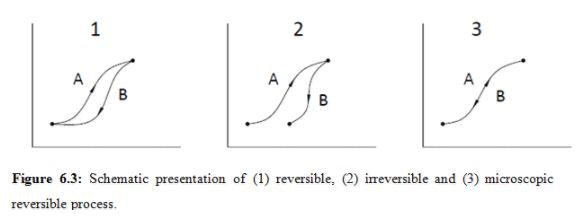In Figure 6.3, A-refers to the forward path and B- refers to the backward path. In Figure 6.3(1), although the forward and backward path follows a different trajectory, initial and final states are identical (same). Thus the process is reversible.

In Figure 6.3(2), A and B follows different trajectory and the initial points are not the same. Hence, it is an irreversible process.

In Figure 6.3(3), The forward (A) and backward (B) exactly follows the same trajectory. Hence it is also reversible. The difference between 1 and 3 is: in 1 although the initial and final states are identical, the intermediate states are different. But for 3, any state (i.e. point) along the trajectory is same in both A and B. Thus, this is known as ‘microscopic reversibility' and 1 is known as ‘macroscopic reversibility'.

In molecular simulation, similar microscopic reversibility is used. We have seen that in Metropolis sampling, the probability of going from an initial state (i) to a final state (f) is: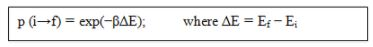We will now derive the above relation using the concept of ‘Principles of detailed balance'. Let us assume a system is at an initial state, i, with energy Ei. The probability of sampling this particular state is given by N(i), which represents the probability density of this state. Now the transition probability from i to f is given by the transition matrix Πij. The acceptance probability is given by acc(i→f).

The overall probability of moving from i to f is given by the product of all the probabilities mentioned above: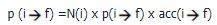(i)

Now consider the reverse process: transition from f to i, and we can write in a similar manner: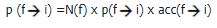(ii)

To satisfy the detailed balance criteria (microscopic reversibility), we can equate equation (I) and (II):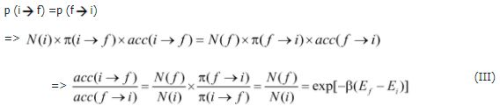In metropolis scheme,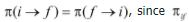, is a symmetric matrix. (i) and (f) are simply expressed by Boltzmann factor, exp(-ßEi) and exp(-ßEf) respectively. Equation (III) essentially gives the overall probability of moving from i to f.

NOTE: In real MC simulation, we do not need to calculate all the quantities in equation (I) and (II). We need to calculate E i and E f only at a particular state of the system (e.g., temperature). This will give us the value of p (i→f) =exp(-ΔE/kBT). Generate a random number, r. If r = p (i→f) → accept the new state, else retain the old state.

Ergodicity: Every accessible point in a configuration space can be reached in a finite number of MC steps from any other point.

159 docs

,

,

,

,

,

,

,

,

,

,

,

,

,

,

,

,

,

,

,

,

,

,

,

,

;